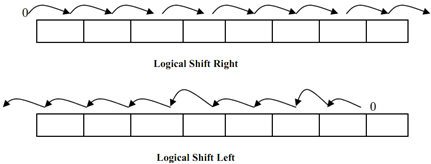## Explain logical shifts with example, Computer Engineering

Assignment Help:

Q. Explain Logical shifts with example?

Logical shifts LOGICAL SHIFT LEFT and LOGICAL SHIFT RIGHT insert zeros to end bit position and other bits of a word are shifted left or right correspondingly. The end bit position is leftmost bit for shift right and rightmost bit position for shift left. Bit shifted out is lost.Figure: Logical Shift

#### Related Discussions:- Explain logical shifts with example

Linked list means node which is linked each other with  a line. It means that every node is connected with another one. Every node of the list hold the reference of the next node.

#### Performance equation of computer system, Performance Equation of computer s...

Performance Equation of computer system: Following equation is frequently used for expressing a computer's performance ability: The CISC approach tries to minimize the

#### Computer architecture, pipelining hazards

pipelining hazards

#### Explain the term - integrity, Explain the term - Integrity In most c...

Explain the term - Integrity In most cases, corporate data should remain unchanged by third parties, so the system should be capable of ensuring that only authorised personn

#### What is a demultiplexer, What is a Demultiplexer ? Ans. Demultiplex...

What is a Demultiplexer ? Ans. Demultiplexer has similar circuit as decoder but here E is obtained as the particular input line, the output lines are similar as decode

#### Predicates - first-order logic, Predicates - first-order logic: First ...

Predicates - first-order logic: First and foremost in first-order logic sentences, because predicates. Hence the indications of that some things are related in some way. So af

#### What are the entities that swapped out of the main memory, What are the ent...

What are the entities that are swapped out of the main memory while swapping the process out of the main memory? All memory space occupied by the process, process's u-area, and

#### Representation in prolog - logic programs, Representation in Prolog - Logic...

Representation in Prolog - Logic Programs: If we justimpose some additional constraints on first-order logic, so than we get to a representation language knowing as logic prog

#### Recent parallel programming models, A model for parallel programming is an ...

A model for parallel programming is an abstraction and is machine architecture independent. A model can be executed on several hardware and memory architectures. There are various

#### Explain concept of temporal parallelism, Concept of Temporal Parallelism  ...

Concept of Temporal Parallelism  In order to make clear what is meant by parallelism inherent in solution of a problem, let's discuss an example of submission of electricity b